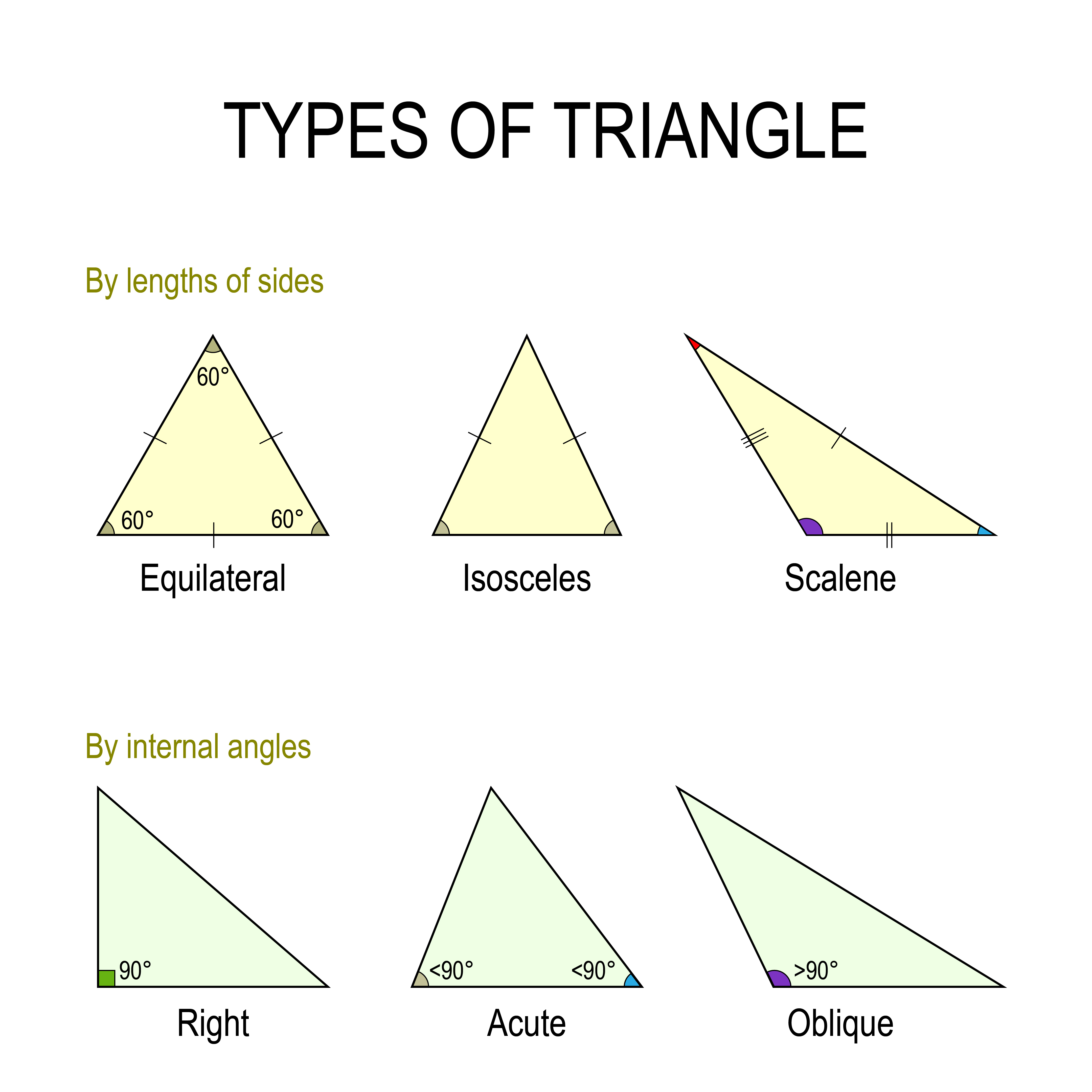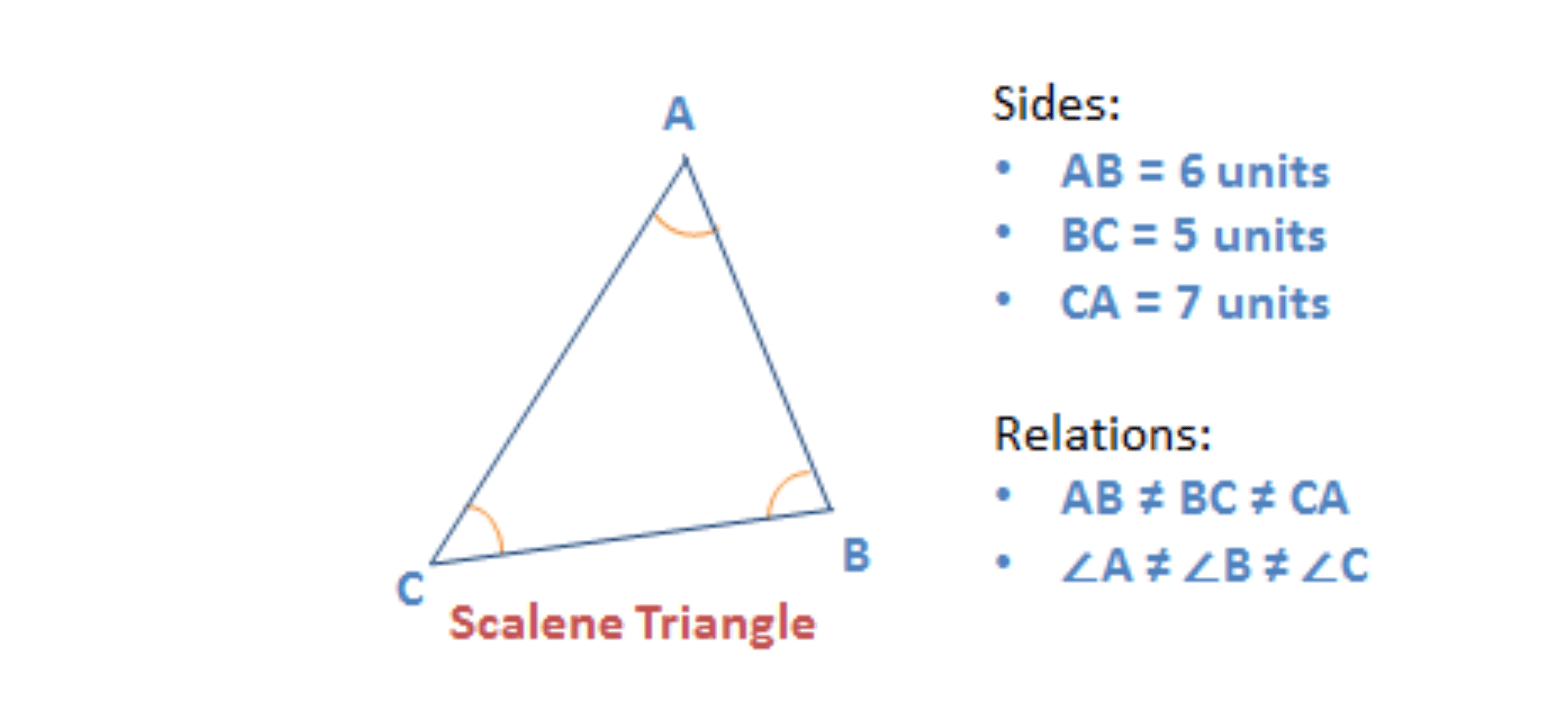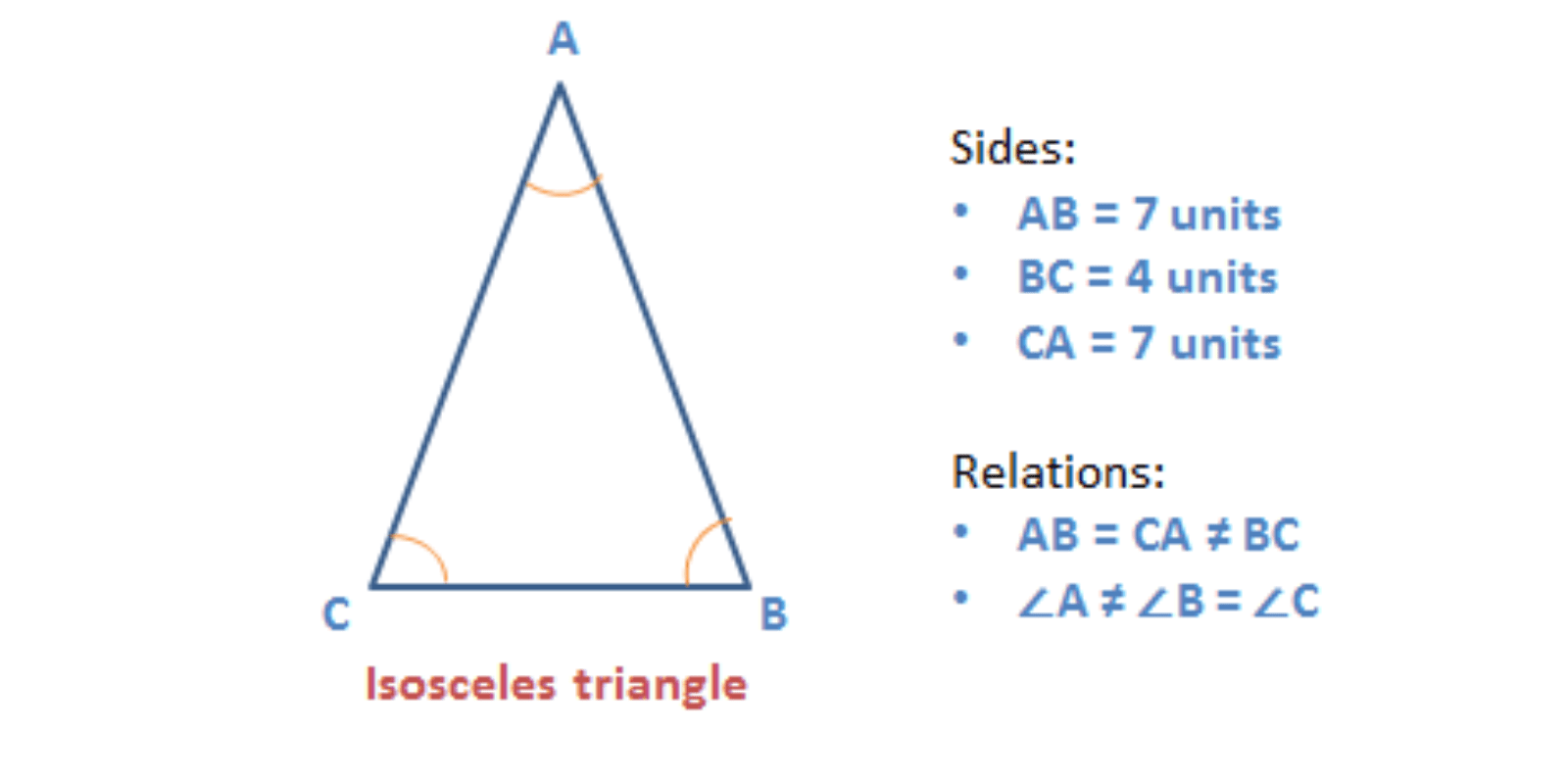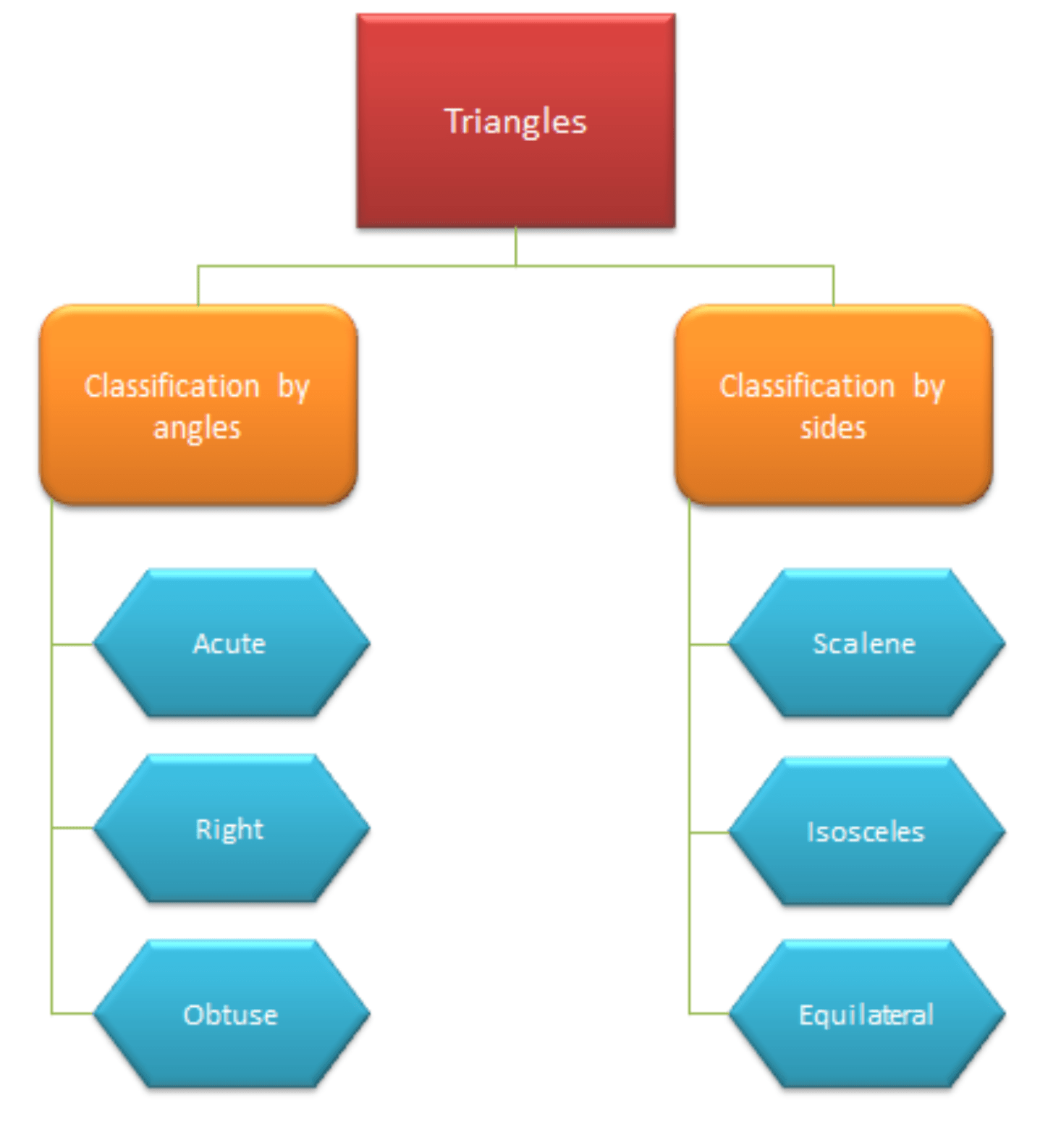A 5 minute read

In this article, we are going to learn about the simplest form of a polygon, a triangle. All polygons can be divided into triangles, or in other words, they are formed by combining two or more triangles. Thus, understanding the basic types and properties of triangles is important.So, let’s begin.

## Definition of a Triangle

As the name suggests, tri – angle is a polygon that has three angles.

So, when does a closed figure has three angles?

• When it has three line segments joined end to end, right?

Thus, we can say that a triangle is a polygon, which has three sides, three angles, and three vertices.

• And, the sum of all three angles of any triangle equals to 1800.

The diagram below is a triangle ABC, and the sum of all its internal angles = 1800

## Properties of triangles: Types of triangleThere are different kinds of triangles. The variations result from the lengths of the sides and the measures of the angles.

### Classification of a triangle by angles:

Based on the angle measurement, triangles are classified into three types:

1. Acute Angled Triangle
2. Right-Angled Triangle
3. Obtuse Angled Triangle

Let us discuss each type in detail.

### Acute Angle TriangleA triangle which has all three angles less than 900 is an acute angle triangle.

• So, all the angles of an acute angle triangle are called acute angles

Given below is an example of an acute angle triangle.

### Right-Angle TriangleA triangle which has one angle that measures exactly 900 is a right-angle triangle.

• The other two angles of a right-angle triangle are acute angles.
• The side opposite to the right angle is the largest side of the triangle and is called

Given below is an example of a right-angle triangle.

In a right-angled triangle, the sum of squares of the perpendicular sides is equal to the square of the hypotenuse.

• This is known as Pythagoras theorem

Vice versa, we can say that if a triangle satisfies the Pythagoras condition, then it is a right-angled triangle.

### Obtuse/Oblique Angle Triangle

A triangle which has one angle that measures more than 900 is an obtuse angle triangle.

Given below is an example of obtuse/oblique angle triangle.## Properties of triangles: Classification of triangles by sides

Based on the length of the sides, triangles are classified into three types:

1. Scalene Triangle
2. Isosceles Triangle
3. Equilateral Triangle

Let us discuss each type in detail.

### Scalene triangleA triangle which has all three sides of different lengths is a scalene triangle.

• Since all the three sides are of different lengths, the three angles will also be different.

Given below is an example of a scalene triangle

### Isosceles triangleA triangle which has two sides of the same length and the third side of a different length is an isosceles triangle.

• The angles opposite the equal sides measure the same.

Given below is an example of a isosceles triangle.

### Equilateral triangleA triangle which has all the three sides of the same length is an equilateral triangle.

• Since all the three sides are of the same length, all the three angles will also be equal.
• Each interior angle of an equilateral triangle = 600

Given below is an example of an equilateral triangle

## Properties of triangles: Flow Diagram## Special cases of right angle triangle

Let’s also see a few special cases of a right-angled triangle

### 45-45-90 triangle

In this triangle,

• Two angles measure 450, and the third angle is a right angle.
• The sides of this triangle will be in the ratio – 1: 1: √2 respectively.
• This is also called an isosceles right-angled triangle since two angles are equal.

### 30-60-90 triangle

In this triangle,

• This is a right-angled triangle, since one angle = 900
• The angles of this triangle are in the ratio – 1: 2: 3, and
• The sides opposite to these angles will be in the ratio – 1: √3: 2 respectively
• This is a scalene right-angled triangle since all three angles are different.

## Summary of Properties of Triangles

Let us discuss some of the important properties of triangles.

• The sum of all interior angles of any triangle is equal to 1800.
• The sum of all exterior angles of any triangle is equal to 3600.
• An exterior angle of a triangle is equal to the sum of its two interior opposite angles.
• The sum of the lengths of any two sides of a triangle is always greater than the length of the third side.
• Similarly, the difference between the lengths of any two sides of a triangle is always less than the length of the third side.
• The side opposite to the smallest interior angle is the shortest side and vice versa.
• Similarly, the side opposite to the largest interior angle is the longest side and vice versa.
• In case of a right-angled triangle, this side is called the hypotenuse
• The height of a triangle is equal to the length of the perpendicular dropped from a vertex to its opposite side, and this side is considered the base.

If you liked this article, you may also like to read the following advanced level articles on triangles

## Area of a triangle

Area of any triangle = ½ * base * height

• Area of a right-angled triangle = ½ * product of the two perpendicular sides

## Question: 1

In an isosceles triangle DEF, if an interior angle ∠D = 1000 then what is the value of ∠F?

1. 200
2. 400
3. 600
4. 800
5. 1000

### Solution

Step 1: Given

• ∆DEF is an isosceles triangle
• ∠D = 1000

Step 2: To find

• The value of ∠F

Step 3: Approach and Working out

• We know that the sum of all interior angles in a triangle = 1800
• Implies, ∠D + ∠E + ∠F = 1800
• ∠E + ∠F = 1800 – 1000 = 800
• Since ∆DEF is an isosceles triangle; two of its angles must be equal.
• And the only possibility is ∠E = ∠F
• Therefore, 2∠F = 800
• Implies, ∠F = 400

Hence the correct answer is Option B.

## Question 2

In a right-angled triangle, ∆ABC, BC = 26 units and AB = 10 units. If BC is the longest side of the triangle, then what is the area of ∆ABC?

1. 120
2. 130
3. 240
4. 260
5. 312

### Solution

Step 1: Given

• ∆ABC is a right-angled triangle
• BC = 26 units
• AB = 10 units
• BC is the longest side of the triangle

Step 2: To find

• The area of triangle ∆ABC

Step 3: Approach and Working out

• We are given that BC is the longest side of the triangle, which implies that BC is the hypotenuse

Thus, according to Pythagoras rule:

• BC2 = AB2 + AC2
• 262 = 102 + AC2
• AC2 = 676 – 100 = 576
• Therefore, AC = 24 units
• We know that the area of a right-angled triangle = ½ * product of the two perpendicular sides = ½ * AB * AC = ½ * 10 * 24 = 120 sq. units

Hence the correct answer is Option A.sPayal Tandon
Co-founder, e-GMAT
Welcome to e-GMAT Support!
I am Payal, Co-Founder of e-GMAT.
Feel free to ask any Query.Thank you for your query.
We will be contacting you soon on